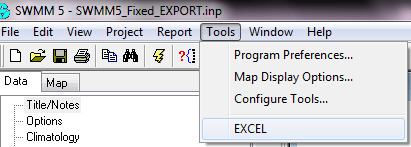SWMM5

# How to Make an Excel Fixed Width File from SWMM 5

How to Make an Excel Fixed Width File from SWMM 5

You can use this macro for MATLAB or some other DOS based program by using the Tools command of SWMM 5 and configuring the EXCEL file option. If you use the EXCEL tool then your SWMM 5 input file will be opened up in EXCEL and you can use this macro to make a fixed format file for SWMM 5. Note, SWMM 5 does not use fixed format but other programs may need it for reading, You adjust the column widths in the macro for your program.Here is the macro, which is placed in Excel using these command:

1. Copy the code above.
2. Open Excel.
3. Alt + F11 to open the Visual Basic Editor.
4. Click Insert-Module from the menu.
5. Paste the code into the window that appears at right.

Option Explicit

Sub CreateFixedWidthFile(strFile As String, ws As Worksheet, s() As Integer)

Dim i As Long, j As Long

Dim strLine As String, strCell As String

'get a freefile

Dim fNum As Long

fNum = FreeFile

'open the textfile

Open strFile For Output As fNum

'loop from first to last row

'use 2 rather than 1 to ignore header row

For i = 1 To ws.Range("a65536").End(xlUp).Row

'new line

strLine = ""

'loop through each field

For j = 0 To UBound(s)

'make sure we only take chars up to length of field (may want to output some sort of error if it is longer than field)

strCell = Left\$(ws.Cells(i, j + 1).Value, s(j))

'add on string of spaces with length equal to the difference in length between field length and value length

strLine = strLine & strCell & String\$(s(j) - Len(strCell), Chr\$(32))

Next j

'write the line to the file

Print #fNum, strLine

Next i

'close the file

Close #fNum

End Sub

'for example the code could be called using:

Sub CreateFile()

Dim sPath As String

sPath = Application.GetSaveAsFilename("SWMM5_Fixed_EXPORT", "Text Files,*.inp")

If LCase\$(sPath) = "false" Then Exit Sub

'specify the widths of our fields

'the number of columns is the number specified in the line below +1

Dim s(15) As Integer

'starting at 0 specify the width of each column for the SWMM5 File, alter the columns if you need more columns in your data input file

s(0) = 40

s(1) = 20

s(2) = 20

s(3) = 20

s(4) = 20

s(5) = 20

s(6) = 20

s(7) = 20

s(8) = 20

s(9) = 20

s(10) = 20

s(11) = 20

s(12) = 20

s(13) = 20

s(14) = 20

s(15) = 20

'for example to use 3 columns with field of length 5, 10 and 15 you would use:

'dim s(2) as Integer

's(0)=5

's(1)=10

's(2)=15

'write to file the data from the activesheet

CreateFixedWidthFile sPath, ActiveSheet, s

End Sub

Categories: SWMM5